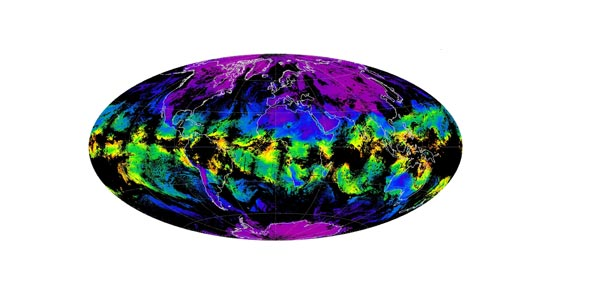# Atmosphere Phenomena Practice Quiz

38 Questions | Total Attempts: 195SettingsUse this quiz as a practice quiz for the Astmosphere Phenomena Test

• 1.
You can calculate the __________________________ of air if you have the mass and the volume.
• A.

Pressure

• B.

Mass

• C.

Density

• 2.
When you are at the top of a mountain, it is easier to lose your breath because there are less ________________________ molecules per cubic meter of air.
• A.

Oxygen

• B.

Nitrogen

• C.

Carbon dioxide

• 3.
The condition of the Earth's atmosphere at a particular time and place is known as _____________________.
• A.

Weather

• B.

Atmosphere

• C.

Water vapor

• 4.
One of the most important features of the atmosphere is that it traps the energy from the Sun, allowing water to exist as a ________________________.
• A.

Solid

• B.

Liquid

• C.

Gas

• 5.
This gas is almost four times greater than the concentration of oxygen in the Earth's atmosphere
• A.

Carbon dioxide

• B.

Argon

• C.

Nitrogen

• 6.
Which of the following is made up of three molecules of oxygen?
• A.

Ozone

• B.

Nitogen oxide

• C.

Sulfuric acid

• 7.
The atmospheric gas ______________________ forms clouds when it condenses.
• A.

Water vapor

• B.

Oxygen

• C.

Nitrogen

• 8.
The layer in the atmosphere where weather occurs is called the
• A.

Trophospere

• B.

Mesosphere

• C.

Thermosphere

• 9.
Instruments used to measure air pressure without the use of liquid are called
• A.

Aneroid thermometers

• B.

Aneroid hydrometers

• C.

Aneroid barometers

• 10.
Almost half of the pollution that comes from humans is caused by _____________________________________________.
• A.

Factories and power plants

• B.

Car vehicle exhaust

• C.

Farming and Construction

• 11.
Photochemical smog results from the interaction of pollutants in the presence of
• A.

Sunlight

• B.

Water

• C.

Air

• 12.
A ________________________ barometer measures air pressure by using a metal chamber containing a "liquid" that is sensitive to pressure changes.
• A.

Aneroid

• B.

Mercury

• C.

Millibar

• 13.
Most natural pollution in the air comes from ______________________
• A.

Forest fires

• B.

Volcanic eruptions

• C.

Burning fossil fuels

• 14.
As altitude increases, density decreases
• A.

True

• B.

False

• 15.
The Aurora Borealis occurs when high energy particles called lunar winds get caught in the Earth's magnetic field.
• A.

True

• B.

False

• 16.
The atmosphere is made of 78% nitrogen and 21% carbon dioxide.
• A.

True

• B.

False

• 17.
Nitrogen oxides react with other air pollutants in the presence of sunlight to form acid rain.
• A.

True

• B.

False

• 18.
The layer where meteoroids burn in the atmosphere is called the Thermosphere.
• A.

True

• B.

False

• 19.
The coldest layer of the atmosphere is the mesosophere; approaching -90*C.
• A.

True

• B.

False

• 20.
Soil erosion, dust storms, and forest fires all account for less air pollution than burning fossil fuels.
• A.

True

• B.

False

• 21.
The envelope of gasses that surround the Earth is called the weather.
• A.

True

• B.

False

• 22.
As the altitude decrease, the density also decreases.
• A.

True

• B.

False

• 23.
Factories produce less air pollution than motor vehicles.
• A.

True

• B.

False

• 24.
The temperature in the atmosphere alternates from later to layer by changing from colder to warmer.
• A.

True

• B.

False

• 25.
Layers in the atmosphere are classified based on their temperature
• A.

True

• B.

False

Related TopicsBack to top